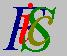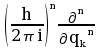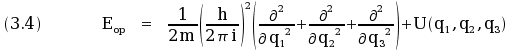Mauro Murzi's pages on Philosophy of Science - Quantum mechanics
Quantum operators

# [3. Schrödinger equations.]

## Quantum operators.

An operator is a rule that transcripts (that is transforms) a given function in another function. Quantum mechanics makes use of operator for translating classical mechanical equations in quantum mechanical equations.

Let u be a classical mechanical quantity. Every classical mechanical quantity is defined by position and momentum, so u is a function of q and p : u = u (q1 , q2 , q3 , p1 , p2 , p3)

In quantum mechanics, an operator uop corresponding to u is defined by means of the following two rules acting on the equation u = u (q1 , q2 , q3 , p1 , p2 , p3).

1. Substitute every occurrences of qk with the operator "multiplication by qk" and every occurrences of f (qk) with the operator "multiplication by f (qk)".
2. Substitute every occurrences of pkn with the operator.

For example, let u be the mechanical energy E given by (3.3). The operator Eop associated to E is:.

Note that in (3.4) the expression U(q1 , q2 , q3) means "multiply by U(q1 , q2 , q3) ".